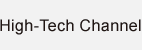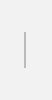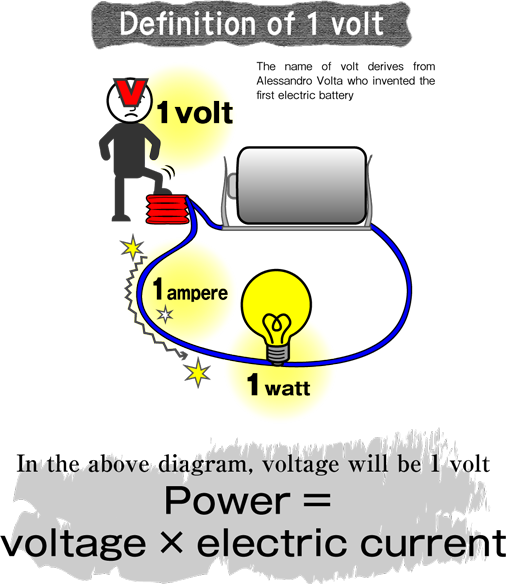### Determining the force at which electric current is pushed out

Symbol: V / Base data: Voltage, potential difference, electromotive force. SI derived unit.
Voltage between 2 points when 1W of power is consumed between 2 points where 1A of electrical current flows

”Voltage” – easy to understand when compared to water pressure

“Volt” is a unit of voltage. Voltage refers to the force at which electricity is pushed out, and is often compared to water pressure. Just as water current becomes larger the greater the water pressure, the higher the voltage the greater the electric current. If power is 1 watt at 1 ampere of electric current, the voltage will be 1 volt.